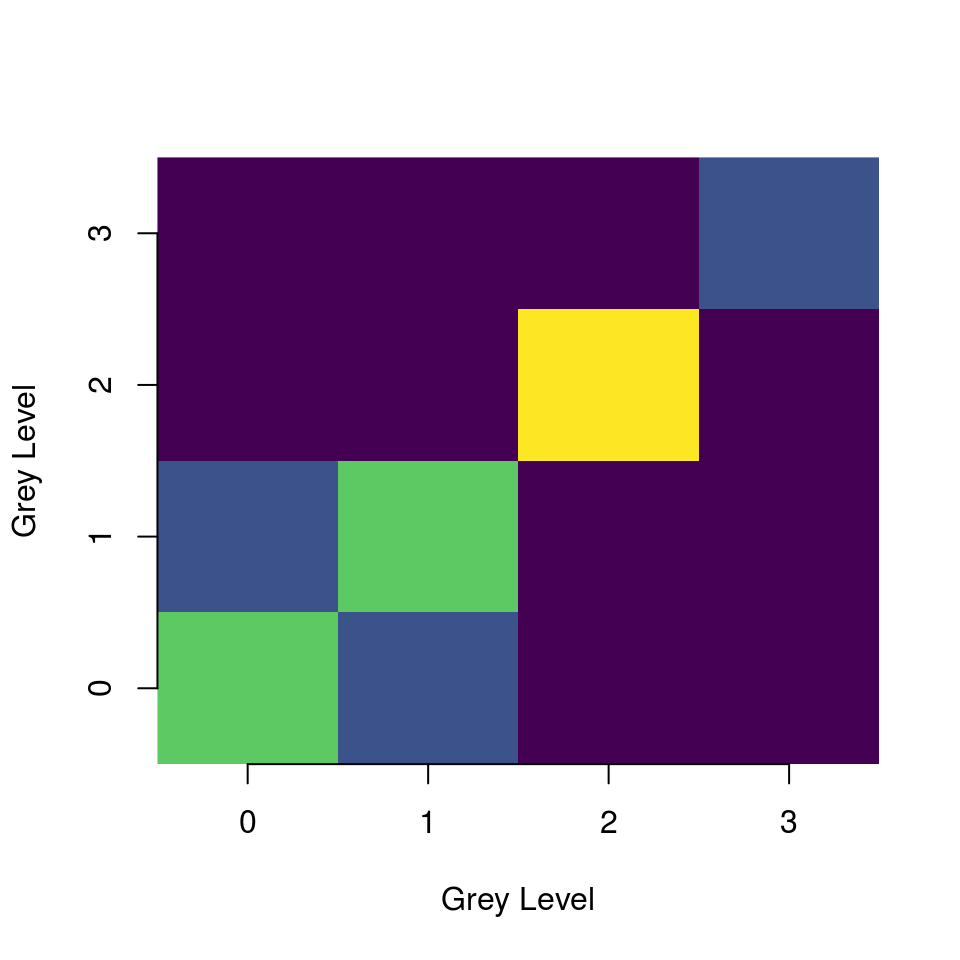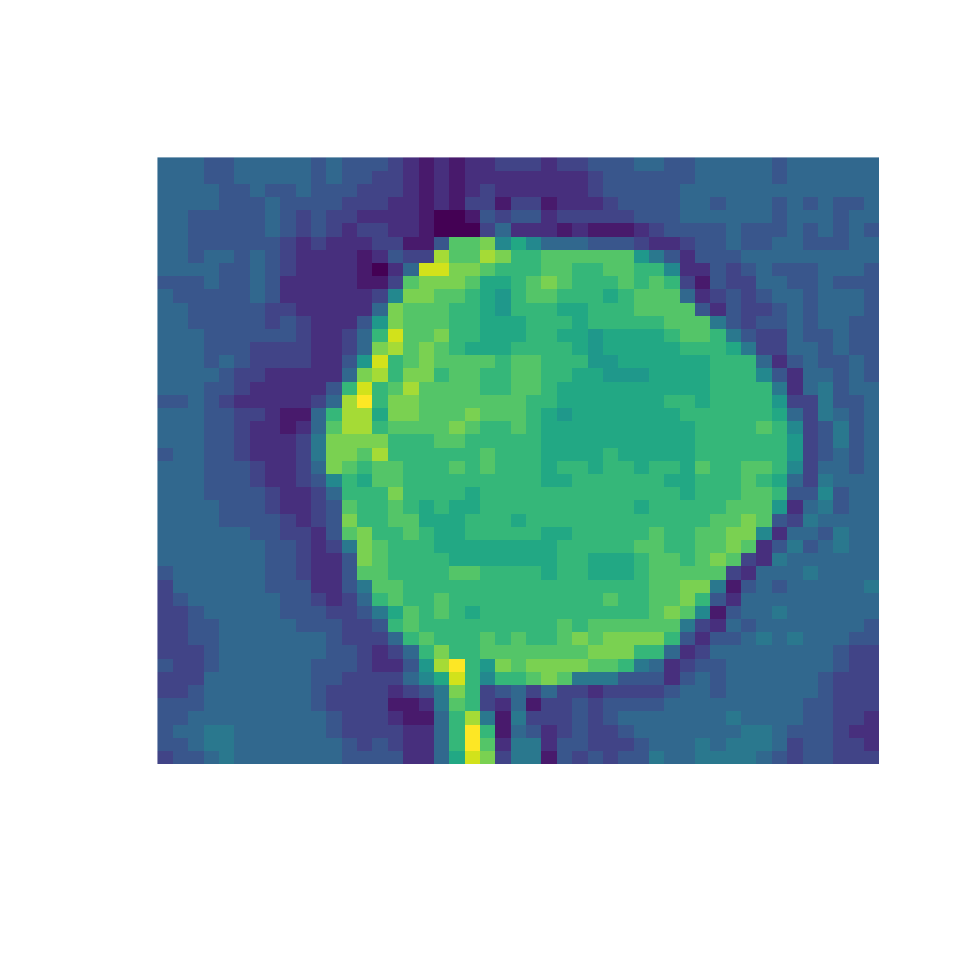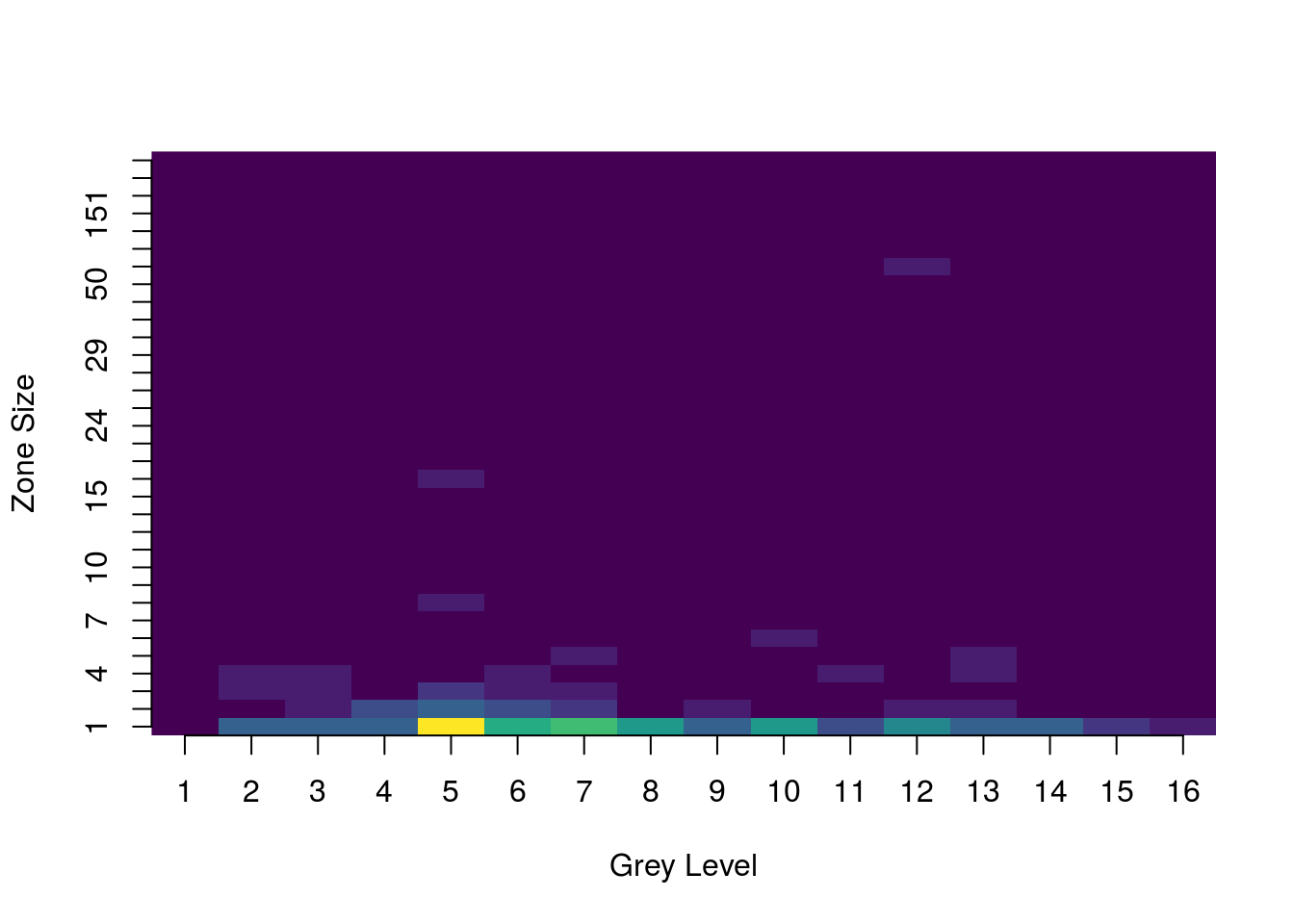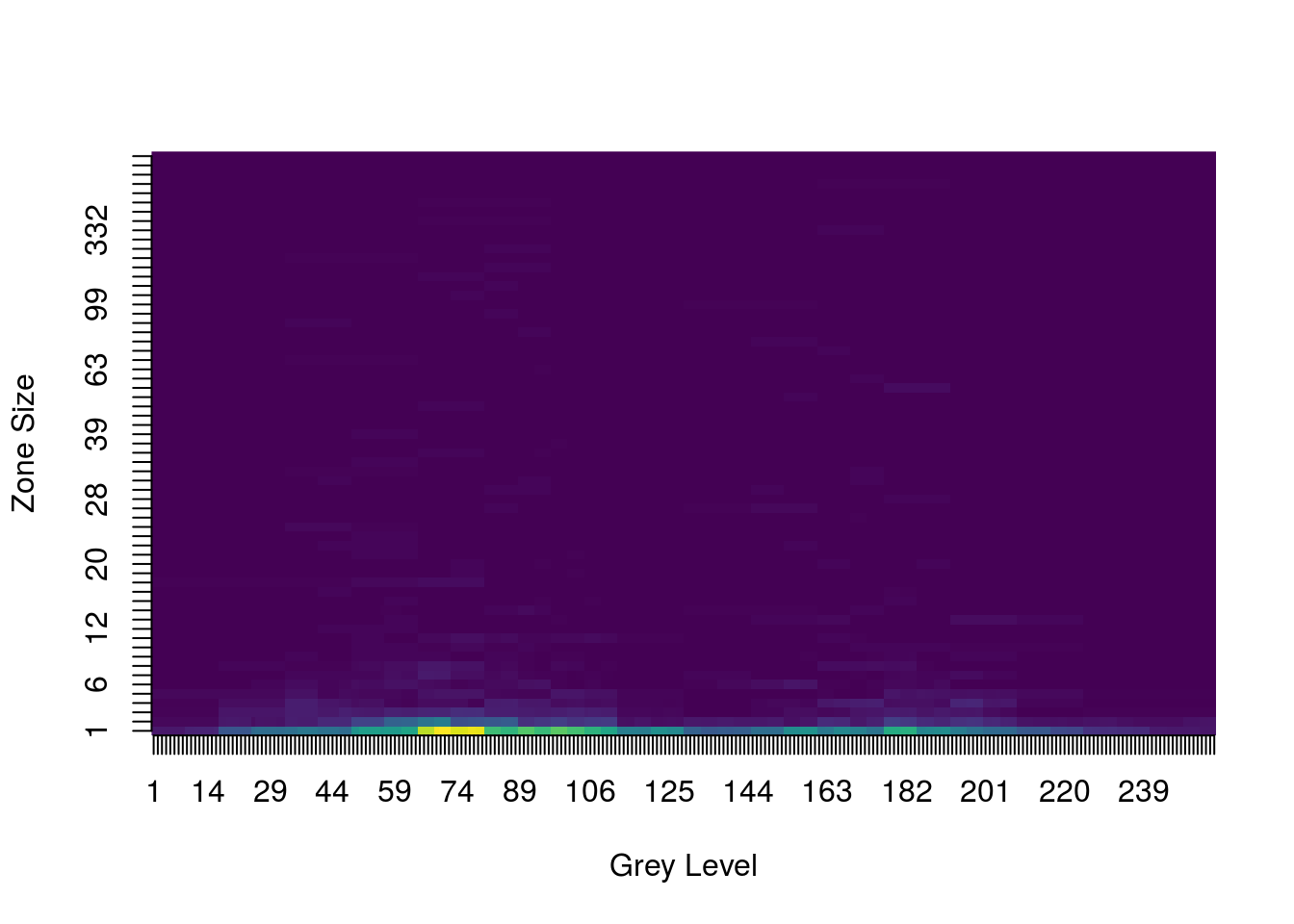Texture analysis is a technique used to quantify the patterns in images that are simple for humans to see, but prove more difficult for computers. The texture analysis features which can be computed using this package can be used as inputs for a classification algorithm, or for visualization of different image properties. In this vignette we will explore the different matrices and features used to quantify texture available in the radiomics package.

This package includes new classes (which inherit from the “matrix” class) for four different types of texture matrices: “grey level co-occurrence” (glcm), “grey level run-length” (glrlm), “grey level size-zone” (glszm), and “multiple grey level size-zone” (mglszm). For each of these matrices a number of features have been defined which characterize the image in question.

library(radiomics)

## Basics

The features calculated by the package can be accessed using the function calc_features on the appropriate class of matrix. For a simple 2D matrix of class “matrix”, the function will calculate the first-order features (described below), for a matrix of class “glcm”, the glcm feature set will be calculated, and so on for the remaining matrix types. The features are returned as a single observation data frame

The most basic possible use of the function is as follows:

test <- matrix(sample(1:10, 25, replace=TRUE), ncol=5)
calc_features(test)

## First order features

Included in the package is a method for calculating several first-order features of images. First-order features are features which rely only on the values of individual pixels in the image, and do not express their relationship to other image pixels. For example, the mean/median/minimum/maximum pixel values in the image.

The first-order features included are as follows:

Feature Argument
Energy calc_energy
Entropy calc_entropy
Kurtosis calc_kurtosis
Mean Deviation calc_meanDeviation
Skewness calc_skewness
Uniformity calc_uniformity
Mean calc_mean
Median calc_median
Maximum calc_max
Minimum calc_min
Variance calc_variance
Root Mean Square calc_RMS
Standard Deviation calc_sd

Arguments can be used to select only certain features for calculation by adding them to the features argument of calc_features:

calc_features(test, features = c("calc_energy", "calc_mean"))

## Grey Level Co-occurrence Matrix (GLCM)

The GLCM is a matrix which considers the spatial relationships between two pixels at a time in the image (the reference and the neighbor pixel). The neighbor pixel can be to the East (0), North-East (45), North (90), or North-West (135) of the reference pixel. The distance between the reference and neighbor pixel can also be chosen.

The matrix is built such that each row represents a single grey value in the image, and each column also represents a single grey value. The entries of the matrix consist of the number of the times each gray level in a reference position occurs with each other gray level in the neighbor position. The matrix is then added to it’s transpose to create a symmetrical matrix

For a more detailed explanation of the GLCM see here or here and for the seminal 1974 paper, see here (Warning: PDF download)

GLCMs can be created using the glcm function, and can be calculated for different angles and distances using the angle and d arguments:

#Load the dataset from the Hallbey tutorial:
data(hallbey)
(hbGLCM <- glcm(hallbey, angle=0, d=1))
## An object of class "glcm"
##            0          1          2          3
## 0 0.16666667 0.08333333 0.04166667 0.00000000
## 1 0.08333333 0.16666667 0.00000000 0.00000000
## 2 0.04166667 0.00000000 0.25000000 0.04166667
## 3 0.00000000 0.00000000 0.04166667 0.08333333

And as with first order features, glcm features can be calculated by using the calc_features function:

calc_features(hbGLCM)

And can be visualized using the image generic:

image(hbGLCM)The available features for the GLCM are as follows:

GLCM features
Feature Argument
Mean glcm_mean
Variance glcm_variance
Auto Correlation glcm_autoCorrelation
Cluster Prominence glcm_cProminence
Cluster Tendency glcm_cTendency
Contrast glcm_contrast
Correlation glcm_correlation
Difference Entropy glcm_differenceEntropy
Dissimilarity glcm_dissimilarity
Energy glcm_energy
Entropy glcm_entropy
Homogeneity1 glcm_homogeneity1
Homogeneity2 glcm_homogeneity2
Inverse Difference Moment (Normalized) glcm_IDMN
Inverse Difference Moment glcm_IDN
Inverse Variance glcm_inverseVariance
Maximum Probability glcm_maxProb
Sum Average glcm_sumAverage
Sum Entropy glcm_sumEntropy
Sum Variance glcm_sumVariance

## Grey Level Run Length Matrix (GLRLM)

Rather than looking at pairs of pixels, the GLRLM looks at runs of pixels. That is, how many pixels of a given grey value occur in a sequence in a given direction.

The rows of the GLRLM represent, again, grey levels in the image. However, the columns now represent lengths of runs, with the entries corresponding to the number of runs of the given length in the image. Let’s look at the example matrix used in the Galloway paper from 1974 which first described the GLRLM.

##      [,1] [,2] [,3] [,4]
## [1,]    0    1    2    3
## [2,]    0    2    3    3
## [3,]    2    1    1    1
## [4,]    3    0    3    0

And the GLRLM:

glrlm(s, angle=0, verbose=F)
## An object of class "glrlm"
##   1 2 3
## 0 4 0 0
## 1 1 0 1
## 2 3 0 0
## 3 3 1 0

With available features:

GLRLM features
Feature Argument
Grey Level Non-uniformity glrlm_GLN
High Grey Level Run Emphasis glrlm_HGLRE
Long Run Emphasis glrlm_LRE
Long Run High Grey Level Emphasis glrlm_LRHGLE
Long Run Low Grey Level Emphasis glrlm_LRLGLE
Low Grey Level Run Emphasis glrlm_LGLRE
Run Length Non-uniformity glrlm_RLN
Run Percentage glrlm_RP
Short Run Emphasis glrlm_SRE
Short Run High Grey Level Emphasis glrlm_SRHGLE
Short Run Low Grey Level Emphasis glrlm_SRLGLE

## Grey Level Size Zone Matrix (GLSZM) and Multiple-GLSZM

The GLSZM looks at zones of 9-connected pixels. That is, how many pixels of a given grey value are connected in a single group.

The rows of the GLSZM represent grey levels in the image, and the columns now represent sizes. Thus the entries correspond to the number of groups of pixels of a given grey value that are a given size.

For the GLSZM let’s look at a more complicated image, that of a tumor, but discretized into only 16 grey levels (using the discretizeImage function included in the package)

discTumor <- discretizeImage(radiomics::tumor, n_grey=16)
image(discTumor, axes=F, col=viridis::viridis(16))And the corresponding GLSZM:

image(glszm(discTumor))The MGLSZM computes a GLSZM for 8 different discretizations of the image (2, 4, 8, 16, 32, 64, 128, and 256 grey levels). It then combines these using a weights based a Gaussian distribution (mean = 0, standard deviation = 1).

The MGLSZM for the tumor image looks like:

image(mglszm(tumor))The GLSZM and MGLSZM use the same feature set:

GLSMZ and MGLSZM features
Feature Argument
Small Area Emphasis glszm_SAE
Large Area Emphasis glszm_LAE
Intensity Variability glszm_IV
Size Zone Variance glszm_SZV
Zone Percentage glszm_ZP
Low Intensity glszm_LIE
High Intensity Emphasis glszm_HIE
Low Intensity Small Area Emphasis glszm_LISAE
High Intensity Small Area Emphasis glszm_HISAE
Low Intensity Large Area Emphasis glszm_LILAE
High Intensity Large Area Emphasis glszm_HILAE

Happy classifying!